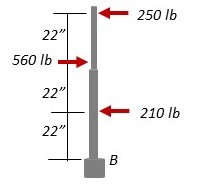# Determine the height h above the base B at which the resultant of the three forces acts.

## Question:

Determine the height h above the base B at which the resultant of the three forces acts.## Torque:

It is a measure of force which causes the object to rotate about an axis.

It is similar to the force as we used in kinematics.

It is a vector quantity.

It depends upon the force and the point of application of force or the distance from the axis where the force is applied.

Torque about any axis is defined as:

{eq}\vec{\tau} = \vec{r} \times \vec{F}\\ \tau = r.F \sin \theta {/eq}

where,

• {eq}\vec{r} {/eq} is the distance vector from the axis where the force is applied.
• {eq}\vec{F} {/eq} is the force applied.

Become a Study.com member to unlock this answer! Create your account

In the figure, if we wish to replace the three forces with one, we need to calculate the net force which will replace these forces.

So, the net force...Torque in Physics: Equation, Examples & Problems

from

Chapter 3 / Lesson 13
31K

After watching this video, you will be able to explain what torque is and use an equation to calculate torque in simple situations. A short quiz will follow.